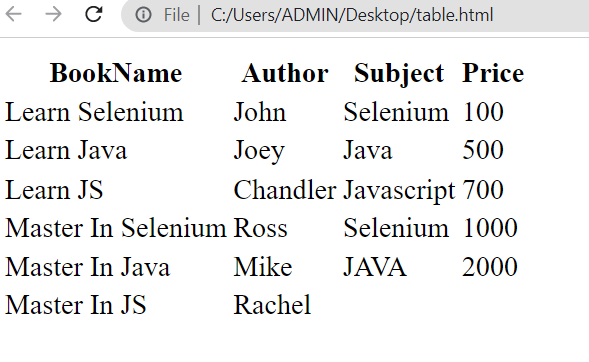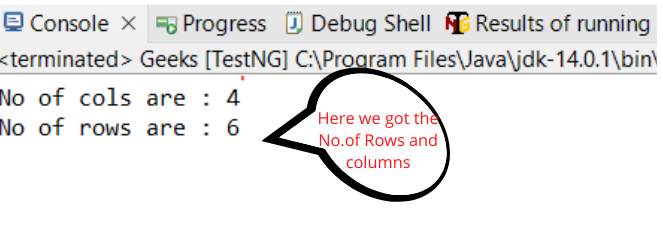GeeksforGeeks App
Open AppBrowser
Continue

# How to Handle Dynamic Web Tables using Selenium WebDriver in Java?

A Dynamic web table is a type of web table where the number of rows and columns are not constant, they keep changing from time to time depending on the requirements or based on the query, i.e. Number of rows and columns is NOT fixed.

### Example of HTML Table

Dynamic Table

The below table is a dynamic web table and the HTML code for the table, This table does not contain even an arrangement of rows and columns like the last row has two columns only, but the other has 4 columns.

## HTML

 `<``html``>``   ``<``head``>``      ``<``style``>``   ````   ``<``body``>``      ``<``table` `name``=``"Table"``>``         ``<``tr``>``            ``<``th``>BookName``            ``<``th``>Author``            ``<``th``>Subject``            ``<``th``>Price``         ````         ``<``tr``>``            ``<``td``>Learn Selenium``            ``<``td``>John``            ``<``td``>Selenium``            ``<``td``>100``         ````         ``<``tr``>``            ``<``td``>Learn Java``            ``<``td``>Joey``            ``<``td``>Java``            ``<``td``>500``         ````         ``<``tr``>``            ``<``td``>Learn JS``            ``<``td``>Chandler``            ``<``td``>Javascript``            ``<``td``>700``         ````         ``<``tr``>``            ``<``td``>Master In Selenium``            ``<``td``>Ross``            ``<``td``>Selenium``            ``<``td``>1000``         ````         ``<``tr``>``            ``<``td``>Master In Java``            ``<``td``>Mike``            ``<``td``>JAVA``            ``<``td``>2000``         ````         ``<``tr``>``            ``<``td``>Master In JS``            ``<``td``>Rachel``         ````      ````   `````

Save the code as “.html”, then you will get an HTML table like below.### Example for fetch no. of rows and columns from Dynamic Table

The main problem that occurs during working with the Dynamic table is, that we cannot predict the number of rows and columns. So in this example, we will use the Selenium web driver to find the number of rows and columns. For computing the number of rows and columns, we require the Xpath of the web table.

Find the X-Path of the Table:

Go to the website, Right-click on the table and select inspect and copy the x-path.

X-Path for Columns:

/html/body/table/tbody/tr/th

X-Path for Rows:

/html/body/table/tbody/tr/td

## Java

 `import` `java.util.List;``import` `org.openqa.selenium.By;``import` `org.openqa.selenium.WebElement;``import` `org.openqa.selenium.chrome.ChromeDriver;``import` `org.testng.annotations.Test;` `public` `class` `Geeks {` `    ``String columnXpath = ``"/html/body/table/tbody/tr/th"``;``    ``String rowXpath = ``"/html/body/table/tbody/tr/td"``;``    ``@Test` `public` `void` `geeksforgeeks()``    ``{``        ``// Please note that with Selenium 4.6.0 version, a``        ``// new feature is added called Selenium Manager With``        ``// Selenium Manager there is no need to use any``        ``// driver, rather Selenium can handle itself.``        ``System.setProperty(``            ``"webdriver.chrome.driver"``,``            ``"C:\\Users\\ADMIN\\Documents\\chromedriver.exe"``);``        ``ChromeDriver driver = ``new` `ChromeDriver();` `        ``// Maximize the browser``        ``driver.manage().window().maximize();` `        ``// Launch Website``        ``driver.get(``            ``"file:///C:/Users/ADMIN/Desktop/table.html"``);` `        ``// Number of columns``        ``List col``            ``= driver.findElements(By.xpath(columnXpath));``        ``System.out.println(``"No of columns : "` `+ col.size());` `        ``// Number of rows``        ``List rows``            ``= driver.findElements(By.xpath(rowXpath));``        ``System.out.println(``"No of rows : "` `+ rows.size());``        ``driver.close();``    ``}``}`

Code Explanation:

We declared the selenium webdriver object “driver” and initialized it to chrome driver, and used the ‘List<webelement>’ list of web element datatype to find the number of columns and rows.

To set up the chrome driver using selenium refer to this article How to open chrome browser using Selenium in Java

Output:My Personal Notes arrow_drop_up
Related Tutorials